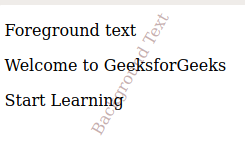Related Articles
How to use text as background using CSS ?
• Last Updated : 22 Apr, 2020

There are some website designs that require using text as the background. This can be easily achieved with CSS by using the following methods.

• Using absolutely positioned element inside relatively positioned element: The absolutely positioned element inside a relative positioned element with absolute element having lower z-index value gives text appears as the background.

Example:

 ` ` `<``html``> ` ` `  `<``head``> ` `    ``<``title``> ` `        ``Using text as background with CSS ` `    `` ` ` `  `    ``<``style``> ` `        ``.container { ` `            ``position: relative; ` `        ``} ` ` `  `        ``.containerbackground { ` `            ``margin: 3rem; ` `            ``position: absolute; ` `            ``top: 0; ` `            ``left: 0; ` `            ``bottom: 0; ` `            ``z-index: -1; ` `            ``transform: rotate(300deg); ` `            ``-webkit-transform: rotate(300deg); ` `            ``color: #c6afaf; ` `        ``} ` `    `` ` ` ` ` `  `<``body``> ` `    ``<``div` `class``=``"container"``> ` `        ``<``div` `class``=``"containerbackground"``> ` `            ``Background Text ` `        `` ` `        ``<``p``>Foreground text ` `        ``<``p``>Welcome to GeeksforGeeks ` `        ``<``p``>Start Learning ` `    `` ` ` ` ` `  ` `

Output:• Using :after pseudo elements: Using :after pseudo elements with :after pseudo elements having lower z-index value make it to appear as background. Use text inside :after pseudo element to make appear text as the background.

Example:

 ` ` `<``html``> ` ` `  `<``head``> ` `    ``<``title``> ` `        ``Using text as background with CSS ` `    `` ` ` `  `    ``<``style``> ` `        ``.bgtext { ` `            ``position: relative; ` `        ``} ` ` `  `        ``.bgtext:after { ` `            ``margin: 3rem; ` `            ``content: "Background text"; ` `            ``position: absolute; ` `            ``transform: rotate(300deg); ` `            ``-webkit-transform: rotate(300deg); ` `            ``color: rgb(187, 182, 182); ` `            ``top: 0; ` `            ``left: 0; ` `            ``z-index: -1; ` `        ``} ` `    `` ` ` ` ` `  `<``body``> ` `    ``<``div` `class``=``"bgtext"``> ` `        ``<``p``>Foreground text ` `        ``<``p``>Welcome to GeeksforGeeks ` `        ``<``p``>Start Learning ` `    `` ` ` ` ` `  ` `

Output:• Using :before pseudo elements: Using :before pseudo elements with :before pseudo elements having lower z-index value make it to appear as background. Use text inside :before pseudo element to make appear text as the background.

Example:

 ` ` `<``html``> ` ` `  `<``head``> ` `    ``<``title``> ` `        ``Using text as background with CSS ` `    `` ` ` `  `    ``<``style``> ` `        ``.bgtext { ` `            ``position: relative; ` `        ``} ` ` `  `        ``.bgtext:before { ` `            ``margin: 3rem; ` `            ``content: "Background text"; ` `            ``position: absolute; ` `            ``transform: rotate(300deg); ` `            ``-webkit-transform: rotate(300deg); ` `            ``color: rgb(187, 182, 182); ` `            ``top: 0; ` `            ``left: 0; ` `            ``z-index: -1; ` `        ``} ` `    `` ` ` ` ` `  `<``body``> ` `    ``<``div` `class``=``"bgtext"``> ` `        ``<``p``>Foreground text ` `        ``<``p``>Welcome to GeeksforGeeks ` `        ``<``p``>Start Learning ` `    `` ` ` ` ` `  `        `

Output :My Personal Notes arrow_drop_up
Recommended Articles
Page :